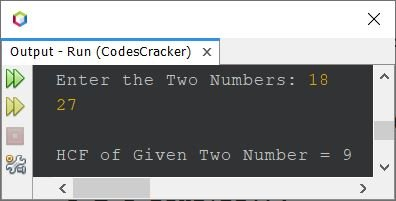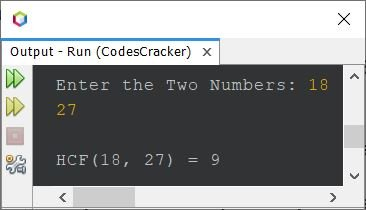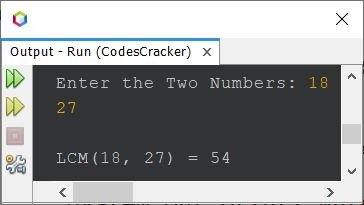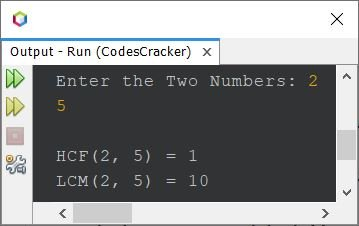# Java Program to Find HCF and LCM of Two Numbers

If you're not aware about, how the HCF and LCF of two numbers gets calculated ?
Refer to, How to Find LCM and How to Find HCF. Now let's move on, and create the program in Java.

Note - HCF stands for Highest Common Factor, whereas LCM stands for Least Common Multiple.

## Program to Find HCF of Two Numbers in Java

The question is, write a Java program to find and print HCF of two numbers. Both the number must be received by user at run-time. The program given below is its answer:

```import java.util.Scanner;

public class CodesCracker
{
public static void main(String[] args)
{
int a, b, gcd;
Scanner s = new Scanner(System.in);

System.out.print("Enter the Two Numbers: ");
a = s.nextInt();
b = s.nextInt();

gcd = a;

while(true)
{
if((a%gcd==0) && (b%gcd==0))
break;
else
gcd--;
}

System.out.println("\nHCF of Given Two Number = " +gcd);
}
}```

The snapshot given below shows the sample run of above Java program on finding and printing the HCF or GCD of two given numbers, with user input 18 and 27That is,

• Factors of 18 are 1, 2, 3, 6, 9, 18
• Factors of 27 are 1, 3, 9, 27

Because 9 is the highest common factor between 18 and 27. Therefore HCF is 9.

In above program, I've assumed one number as gcd (HCF) of two numbers. And then used a loop that always evaluates to be True, until we got a number that divides both the number.

The above program can also be created in this way. Since, HCF deals with highest common factor. Therefore, we need to initialize the smaller number to gcd initially.

```import java.util.Scanner;

public class CodesCracker
{
public static void main(String[] args)
{
int a, b, hcf;
Scanner s = new Scanner(System.in);

System.out.print("Enter the Two Numbers: ");
a = s.nextInt();
b = s.nextInt();

if(a<b)
hcf = a;
else
hcf = b;

while(true)
{
if((a%hcf==0) && (b%hcf==0))
break;
else
hcf--;
}

System.out.println("\nHCF(" +a+ ", " +b+ ") = " +hcf);
}
}```

Here is its sample run with same user input as of previous program's sample run:### Find HCF of Two Numbers using for Loop

The above program can be created in way to use for loop, instead of while. This program also uses conditional operator to make the program shorter.

```import java.util.Scanner;

public class CodesCracker
{
public static void main(String[] args)
{
int hcf, a, b;
Scanner s = new Scanner(System.in);

System.out.print("Enter the Two Numbers: ");
a = s.nextInt();
b = s.nextInt();

for(hcf=(a<b)?a:b; true; hcf--)
{
if((a%hcf==0) && (b%hcf==0))
break;
}

System.out.println("\nHCF(" +a+ ", " +b+ ") = " +hcf);
}
}```

You'll get the same output as of previous program.

## Program to Find LCM of Two Numbers in Java

LCM deals with Multiple, the least common multiple. This program find and prints LCM of two given numbers entered by user.

```import java.util.Scanner;

public class CodesCracker
{
public static void main(String[] args)
{
int a, b, hcf, lcm;
Scanner s = new Scanner(System.in);

System.out.print("Enter the Two Numbers: ");
a = s.nextInt();
b = s.nextInt();

if(a>b)
lcm = a;
else
lcm = b;

while(true)
{
if((lcm%a==0) && (lcm%b==0))
break;
else
lcm++;
}

System.out.println("\nLCM(" +a+ ", " +b+ ") = " +lcm);
}
}```

Here is its sample run, with again same user input as of previous program:That is,

• Multiples of 18 are 18, 36, 54, ...
• Multiples of 27 are 27, 54, ...

Because 54 is the least common multiple of 18 and 27. Therefore LCM is 54.

## Find HCF and LCM of Two Numbers in Java

This program is basically the combined version, as it prints both LCM and HCF (GCD) of any two given numbers.

```import java.util.Scanner;

public class CodesCracker
{
public static void main(String[] args)
{
int x;
Scanner s = new Scanner(System.in);

System.out.print("Enter the Two Numbers: ");
int a = s.nextInt();
int b = s.nextInt();

int numOne = a;
int numTwo = b;

while(b!=0)
{
x = b;
b = a%b;
a = x;
}

int hcf = a;
int lcm = (numOne*numTwo)/hcf;

System.out.println("\nHCF(" +numOne+ ", " +numTwo+ ") = " +hcf);
System.out.println("LCM(" +numOne+ ", " +numTwo+ ") = " +lcm);
}
}```

The sample run with user input 2 and 5 is shown in the snapshot given below:#### Same Program in Other Languages

Java Online Test

« Previous Program Next Program »